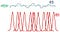# Is Shot Noise Also White Noise?

In Chapters 9 and 11 of Intermediate Physics for Medicine and Biology, Russ Hobbie and I discuss shot noise.

9.8.1 Shot Noise

The first (and smallest) limitation [on our ability to measure current] is called shot noise. It is due to the fact that the charge is transported by ions that move randomly and independently through the channels….

11.16.2 Shot Noise

Chapter 9 also mentioned shot noise, which occurs because the charge carriers have a finite charge, so the number of them passing a given point in a circuit in a given time fluctuates about an average value. One can show that shot noise is also white noise [my italics].

How does one show that shot noise is white noise (independent of frequency)? I’m going to follow Lou DeFelice’s explanation in his book Introduction to Membrane Noise (cited in IPMB). I won’t give a rigorous proof. Instead, I’ll first state Campbell’s theorem (without proving it), and then show that the whiteness of shot noise is a consequence of that theorem.

To start, I’ll quote DeFelice, but I will change the names of a few variables.

Suppose N impulses i(t) arrive randomly in the time interval T. The sum of these will result in a random noise signal I(t). This is shown qualitatively in Figure 78.1.

Below is my version of Fig. 78.1.A diagram illustrating the sum of N impulses, i(t), each shown in red, arriving randomly in the time interval T. The blue curve represents their sum, I(t), and the green dashed line represents the average, . Adapted from Fig. 78.1 in Introduction to Membrane Noise by Louis DeFelice.

DeFelice shows that the average of I(t), which I’ll denote <I(t)>, is

Here he lets T and N both be large, but their ratio (the average rate that the impulses arrived) remains finite.

He then shows that the variance of I( t), called σI2, is

Finally, he writes

In order to calculate the spectral density of I(t) from i(t) we need Rayleigh’s theorem [also known as Parseval’s theorem]…

where î(f) is the Fourier transform of i(t)[and f is the frequency].

He concludes that the spectral density SI(f) is given by

These three results (for the average, the variance, and the spectral density) constitute Campbell’s theorem.

Now, let’s analyze shot noise by using Campbell’s theorem assuming the impulse is a delta function (zero everywhere except at t = 0 where it’s infinite). Set i( t) = q δ( t), where q is the charge of each discrete charge carrier.

First, the average < I( t)> is simply Nq/ T, or the total charge divided by the total time.

Second, the variance is the integral of the delta function squared. When any function is multiplied by a delta function and then integrated over time, you get that function evaluated at time zero. So, the integral of the square of the delta function gives the delta function itself evaluated at zero, which is infinity. Yikes! The variance of shot noise is infinite.

Third, to get the spectral density of shot noise we need the Fourier transform of the delta function.

The key point is that SI(f) is independent of frequency; it’s white.

DeFelice ends with

This [the expression for the spectral density] is the formula for shot noise first derived by Schottky (1918, pp. 541–567) in 1918. Evidently, the variance defined as

is again infinite; this is a consequence of the infinitely small width of the delta function.

As DeFelice reminds us, shot noise is white because the delta function is infinitely narrow. As soon as you assume i(t) has some width (perhaps the time it takes for a charge to cross the membrane), the spectrum will fall off at high frequencies, the variance won’t be infinite (thank goodness!), and the noise won’t be white. The bottom line is that shot noise is white because the Fourier transform of a delta function is a constant.

Perhaps you’re thinking I haven’t helped you all that much. I merely changed your question from “why is shot noise white” to “how do I prove Campbell’s theorem.” You have a point. Maybe proving Campbell’s theorem can be the story of another post.

I met Lou DeFelice in 1984, when I was a graduate student at Vanderbilt University and he came to give a talk. In the summer of 1986, my PhD advisor John Wikswo and I traveled to Emory University to visit DeFelice and Robert DeHaan. During that trip, Wikswo and I were walking across the Emory campus when Wikswo decided he knew a short cut (he didn’t). He left the sidewalk and entered a forest, with me following behind him. After what seemed like half an hour of wandering through a thicket, we emerged from the woods at a back entrance to the Yerkes Primate Research Center. We’re lucky we weren’t arrested.

DeFelice joined the faculty at Vanderbilt in 1995, and we both worked there in the late 1990s. He was a physicist by training, but spent most of his career studying electrophysiology. Sadly, in 2016 he passed away.

Originally published at http://hobbieroth.blogspot.com.

Professor of Physics at Oakland University and coauthor of the textbook Intermediate Physics for Medicine and Biology.